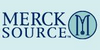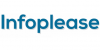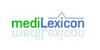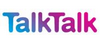subtraction[n] - an arithmetic operation in which the difference between two numbers is calculated 2. [n] - the act of subtracting (removing a part from the whole)
Found on http://www.webdictionary.co.uk/definition.php?query=subtraction

SubtractionSymbol : -The binary operation of finding the difference between two quantities. In arithmetic, subtraction is not commutative, i.e. , nor associative, .The identity element is 0 only when it is on the right hand side, i.e. .
Found on http://www.diracdelta.co.uk/science/source/s/u/subtraction/source.html

SubtractionSub·trac'tion noun [ Latin subtractio a drawing back. See Subtract , and confer Substraction .] 1. The act or operation of subtracting or taking away a part. 2. (Math.) The taking of a lesser number or quantity from a greater of the same kind or denominatio...
Found on http://www.encyclo.co.uk/webster/S/229

subtraction1. The act or operation of subtracting or taking away a part. ... 2. <mathematics> The taking of a lesser number or quantity from a greater of the same kind or denomination; an operation for finding the difference between two numbers or quantities. ... 3. The withdrawing or withholding from a person of some right to which he is entitled by la...
Found on http://www.encyclo.co.uk/local/20973

subtractiondeduction noun the act of subtracting (removing a part from the whole); `he complained about the subtraction of money from their paychecks`
Found on https://www.encyclo.co.uk/local/20974

subtractionnoun an arithmetic operation in which the difference between two numbers is calculated; `the subtraction of three from four leaves one`; `four minus three equals one`
Found on https://www.encyclo.co.uk/local/20974

subtraction(sub-trak´shәn) the photographic or electronic removal of overlying structures from radiologic images.
Found on http://www.encyclo.co.uk/local/21001

Subtraction• (n.) The taking of a lesser number or quantity from a greater of the same kind or denomination; an operation for finding the difference between two numbers or quantities. • (n.) The act or operation of subtracting or taking away a part. • (n.) The withdrawing or withholding from a person of some right to which he is entitled by law...
Found on http://thinkexist.com/dictionary/meaning/subtraction/

subtraction(from the article `arithmetic`) Subtraction has not been introduced for the simple reason that it can be defined as the inverse of addition. Thus, the difference of two numbers ... Vector subtraction is defined by A B = A + (B), where the vector B has the same magnitude as B but the opposition direction. The idea is illus...
Found on http://www.britannica.com/eb/a-z/s/179

subtractionsubtraction, fundamental operation of arithmetic; the inverse of addition. If a and b are real numbers (see number), then the number a-b is that number (called the difference) which when added to b (the subtractor) equals a (the subtrahend). In terms of addition the symbol -b is called the additive ...

subtractionType: Term Pronunciation: sŭb-trak′shŭn Definitions: 1. A technique used to enhance detectability of opacified anatomic structures on radiographic or scintigraphic images; a negative of an image made before introduction of contrast medium or radionuclide is photographically or electronically removed from a later image; commonly used...
Found on http://www.medilexicon.com/medicaldictionary.php?t=86131

subtractionTaking one number or quantity away from another, or finding the difference between two quantities; it is one of the four basic operations of arithmetic. Subtraction is neither commutative: a - bb - a nor associative: a - (...
Found on http://www.talktalk.co.uk/reference/encyclopaedia/hutchinson/m0036920.html

SubtractionSubtraction is a mathematical operation that represents the operation of removing objects from a collection. It is signified by the minus sign (−). For example, in the picture on the right, there are 5 − 2 apples—meaning 5 apples with 2 taken away, which is a total of 3 apples. Therefore, 5 − 2 = 3. Besides counting fruits, subtraction can...
Found on http://en.wikipedia.org/wiki/Subtraction
No exact match found